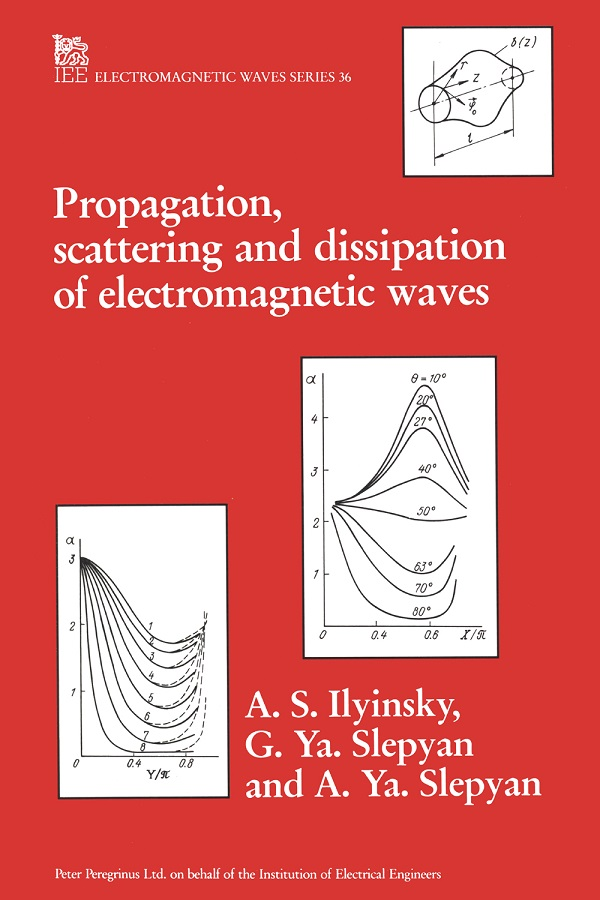In stock
Limited Quantity: 1 in stock

IET Digital Library
This title is available electronically
in the IET Digital Library# Propagation, Scattering and Diffraction of Electromagnetic Waves

##### by A.S. Ilyinski, G.Ya. Slepyan, A.Ya. Slepyan

This book describes new, highly effective, rigorous analysis methods for electromagnetic wave problems. Examples of their application to the mathematical modelling of micros trip lines, corrugated flexible waveguides, horn antennas, complex-shaped cavity resonators and periodic structures are considered.

Special attention is paid to energy dissipation effects. Various physical models and methods of analysis of dissipation are described and approximate formulas and computer-based calculation results for dissipation characteristics are given and compared with experimental data. Ways of decreasing dissipation in waveguides and resonators are discussed.

The book will be of interest to physicists and engineers working on the theory and design of microwave and millimetre-wave components and devices. Designers in microwave engineering will find here all the information they need for choosing the correct waveguide (resonator) for a stipulated dissipation characteristic. The numerical algorithms and formulas can be directly applied to CAD systems. The book is also relevant for students of electromagnetism and microwave circuits.

A.S. Ilyinski is a Professor in the Department of Computational Mathematics and Cybernetics and is Head of the Computational Electrodynamics Laboratory at Moscow State University. He obtained the Doctor of Physical and Mathematical Sciences degree in 1974. His research interests lie in the mathematical modelling of electromagnetic wave scattering, irregular waveguides, microstrip theory, antennas and antenna arrays.

G.Ya. Slepyan is a leading research scientist at the Institute of Nuclear Problems at Belarus State University, Minsk. In 1988 he obtained the Doctor of Physical and Mathematical Sciences degree from Kharkov State University. His research areas include electromagnetic field theory, microwave engineering, free-electron lasers, radiation and scattering in nonlinear media.

A.Ya. Slepyan is a senior research scientist at the Minsk Radioengineering Institute. He obtained the Candidate of Physical and Mathematical Sciences degree in 1985 from the Belarus State University. His research interests are in the scattering and diffraction of electromagnetic waves, mathematical modelling and CAD of antennas and microwave circuits.

Publication Year: 1993

Pages: 288

ISBN-13: 978-0-86341-283-7

Format: HBK